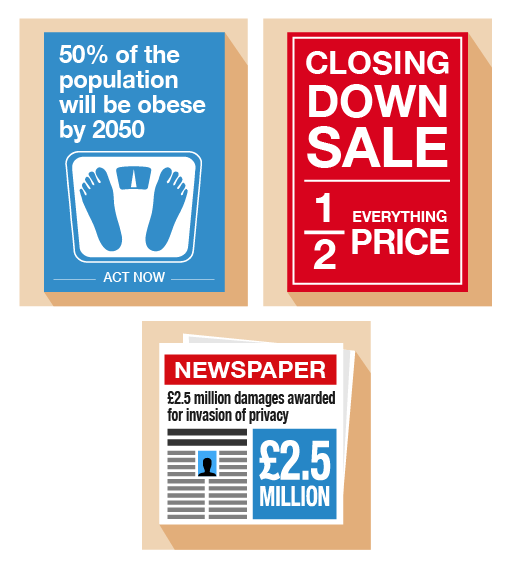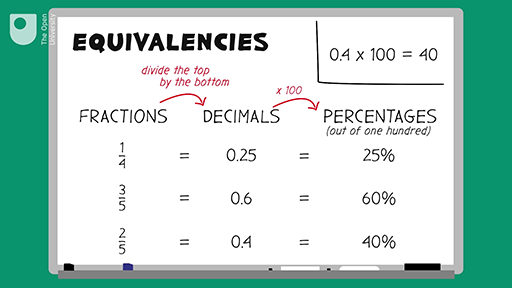Everyday maths 1 (Wales)

Start this free course now. Just create an account and sign in. Enrol and complete the course for a free statement of participation or digital badge if available.

Free course

# 6 Equivalencies between fractions, decimals and percentages

Fractions, decimals and percentages are different ways of saying the same thing. It’s an important skill to learn about the relationships (or ‘equivalencies’) between fractions, decimals and percentages to make sure you are getting the better deal.Figure 31 Looking at equivalencies

Here are some common equivalencies. Try to memorise them – you will come across them a lot in everyday situations:

10% = = 0.1

20% = = 0.2

25% = = 0.25

50% = = 0.5

75% = = 0.75

100% = 1 = 1.0

Look at the following example. If you can identify equivalences, they’ll make it easier to make simple calculations.

## Example: Mine’s a half

What is 50% of £200?

### Method

Since 50% is the same as , so:

50% of £200 = of £200 = £100

Refer to the common equivalencies above (if you need to) to help you with the following activity. Remember to check your answers once you have completed the questions.

## Activity 36: Looking for equivalencies

1. What is 0.75 as a fraction?
2. If you walked 0.25 km each day, what fraction of a kilometer have you walked?
3. House prices have increased by in the last five years. What is this increase as a percentage?
4. A DIY shop is holding a ‘50% off’ sale on kitchens. What is this discount as a fraction?
5. You buy an antique necklace for £3 000. After ten years, its value increases by 20%. What is this increase as a decimal?
6. A headline reads ‘Number of Welsh speakers predicted to rise by 10%’. What is this rise a fraction?
7. What percentage of an hour is 15 minutes?

1. 0.75 as a fraction is .
2. 0.25 is the same as , so you will have walked of a kilometre.
3. is the same as 50%, so the increase is 50%.
4. 50% is the same as , so the discount as a fraction is .
5. 20% is the same as 0.2, so the increase as a decimal is 0.2.
6. 10% is the same as , so according to the headline, the number is predicted to rise by .
7. Think of this as a fraction first: 15 minutes is a quarter () of an hour. is the same as 25%, so 15 minutes is 25% of an hour.

If you find that you are struggling to understand how to convert, please look at the following resource:Skip transcript

#### Transcript

In this video, you’re going to look at equivalent fractions, decimals and percentages. First, let’s look at turning fractions into decimals. You have the fraction one quarter. To turn this into a decimal, you need to divide the top of the fraction by the bottom. 1 divided by 4 equals 0.25. Therefore, 0.25 is the equivalent decimal.

Let’s have a look at another fraction, three fifths. (Remember, divide the top of the fraction by the bottom.) 3 divided by 5 equals 0.6. So 0.6 is the equivalent decimal.

Now let’s try one last fraction see if you can calculate it before the answer is revealed! Two fifths: divide the top by the bottom. 2 divided by 5 equals 0.4. So the decimal is 0.4.

Now let’s use the decimals that we’ve calculated to find the percentage equivalent. A percentage is out of 100. Remember, ‘cent’ means 100. So to turn a decimal into a percentage, you need to multiply the decimal by 100.

For example, 0.25 times 100 equals 25. This is 25%.

0.6 times 100 equals 60, 60%.

0.4 times 100 equals 40. This is 40%.

Now, looking at the table, you can see how fractions, decimals and percentages relate to each other. You just need to remember the basic rules of turning a fraction into a decimal: you divide the top by the bottom, then, once you have the decimal, you can convert to per cent by multiplying by 100, remembering that ‘cent’ means 100.

Thank you for watching. Now you have a go.

End transcript

Interactive feature not available in single page view (see it in standard view).

## Summary

Knowing the common equivalencies between fractions, decimals and percentages is important when trying to compare discounts when shopping or choosing a tariff when paying your bills.

FSM_1_CYMRU#### Live in Wales? Free your ambition with a paid part-time course with the OU in Wales.

With grants of up to £4,500* to help with living costs, and tuition fee loans to cover course fees,
now's the time to take that next step.

#### Find out more

*Eligibility rules apply for financial support.